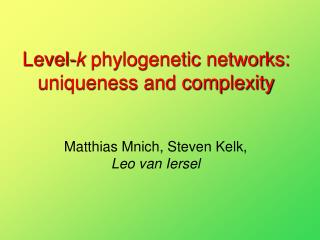# Level- k phylogenetic networks: uniqueness and complexity - PowerPoint PPT PresentationDownload PresentationLevel- k phylogenetic networks: uniqueness and complexity

Level- k phylogenetic networks: uniqueness and complexity
Download Presentation## Level- k phylogenetic networks: uniqueness and complexity

- - - - - - - - - - - - - - - - - - - - - - - - - - - E N D - - - - - - - - - - - - - - - - - - - - - - - - - - -
##### Presentation Transcript

1. Level-k phylogenetic networks: uniqueness and complexity Matthias Mnich, Steven Kelk, Leo van Iersel

2. Phylogenetic networks root A level-k phylogenetic network is a directed acyclic graph where every biconnected component contains at most k recombination vertices. split vertex • Split vertex:indegree 1, outdegree 2 • Recombination vertex: indegree 2, outdegree 1 recombination vertex leaf

3. Example: a level-2 network A level-k phylogenetic network is a directed acyclic graph where every biconnected component contains at most k recombination vertices. • blue = biconnected component • red = recombination vertex

4. Triplets ab|c N A triplet ab|c is consistent with a phylogenetic network Nif N contains a subdivision of ab|c.

5. Triplets ab|c N A triplet ab|c is consistent with a phylogenetic network Nif N contains a subdivision of ab|c.

6. Constructing networks from triplets • ab|c, ac|b, ad|b, bd|a, bd|c, ad|c and ac|d are consistent with this network • bc|a, ab|d, cd|b, bc|d and cd|a are not

7. Consistent level-k network (CL-k) Input: set of triplets T Output: level-k network consistent with T, if one exists. Maximum consistent level-k network (MCL-k) on dense triplet sets Input:dense set of triplets T Output: level-k network N that maximises the number of triplets in T consistent with N. A triplet set is dense if it contains at least one triplet for each combination of three leaves

8. Previous work Remember: k is the level of the networks. Note: a level-0 network is a phylogenetic tree.

9. New results • A level-k network (Nk) that is uniquely defined by its triplets • CL-k is NP-hard for all k • MCL-k is NP-hard for all k, even for dense triplet sets

10. Summary

11. 2. CL-k is NP-hard for all k • Problem:Set Splitting • Input: set S = {s1, …, sn} and collection C = {C1, …, Cm} of cardinality-3 subsets of S. • Question: can S be partitioned into S1 and S2 (a set splitting) such that Cj is not a subset of S1 and not of S2 for all j? REDUCTION FROM: TO: • Problem:Consistent level-k network (CL-k) • Input: set of triplets T. • Question: does there exist a level-k network consistent with T?

12. Start with all triplets consistent with Nk. • For each set Cj={sa,sb,sc} add leavessaj, sbj and scj. • Add triplets that force these leaves togo to one of the red sides. • Add triplets saj r1|sbj, sbj r1|scj and scj r1|saj,which make sure that each set is split. Nk

13. Suppose that set Cj={sa,sb,sc} is not split, i.e. saj, sbj and scj are on the same side. • Then the triplet saj r1|sbj means that saj is below sbj • And the triplet sbj r1|scj means that sbj is below scj • And the triplet scj r1|saj means that scj is below saj So saj r1|sbj, sbj r1|scj and scj r1|saj make it impossible that saj, sbj and scj are all on the same side. contradiction

14. Example • Instance of Set Splitting:C1 = {s1,s3,s4},C2 = {s2,s3,s4},C3 = {s1,s2,s4}. • For C1 we add leaves For C2 we add leavesFor C3 we add leaves • The tripletsenforce that goes to one of the red sides. Nk

15. Example • The tripletsmake sure that C1 is split overthe two red sides. • The tripletsenforce that areon the same side. • In this example there exists aset splitting S1={s1,s3}, S2={s2,s4}. Nk

16. 3. MCL-k is NP-hard for all k, even for dense triplet sets REDUCTION FROM: • Problem:Feedback Arc Set • Input: directed graph G=(V,A) • Output: smallest set A’ of arcs such that G’=(V, A \ A’) is acyclic. TO: • Problem: Maximum consistent level-k network (MCL-k) on dense triplet sets • Input: dense set of triplets T • Output: level-k network N that maximises the number of triplets in T consistent with N.

17. Example for level-2 BigN2

18. Example for level-2 BigN2

19. Start with all triplets consistent with BigN2. • Add triplets that enforce the encoding leaves (u,v,w and q) to go to the red side. • For each arc (v,u) of G add a triplet xu|v, requesting that“v is above u”

20. The arcs corresponding to triplets that are not satisfied form a feedback arc set of G. • Triplet corresponding to arc (w,v) is not satisfied sincew is not “above” v. • Removing (w,v) makes G acyclic because all arcs go to a vertex that is lower on the red path.

21. Summary